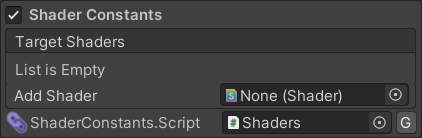The procedural `Shaders` script contains constants corresponding to the IDs of and properties and keywords in the shaders you add to the list in the Weaver Window so that instead of `material.SetTexture("_OcclusionMap", texture)` you can use `material.SetTexture(Shaders.Standard._OcclusionMap, texture)`.

If you want to release a shader to the public which users may want to control via code, feel free to use this system to generate a script to release with the shader.

# Scenes PanelThe Scenes panel in the Weaver Window allows the procedural script to be entirely disabled and allows you to specify which shaders you want it to include.

# Sample Output

``````// This file was procedurally generated by Weaver. Any modifications will be overwritten.

#pragma warning disable // All.

namespace Weaver
{
/// <summary>This class was procedurally generated by Weaver.</summary>
{
/// <summary>Standard</summary>
public static class Standard
{
public const string Name = "Standard";

/// <summary>Color [Color]</summary>

/// <summary>Albedo [2D Texture]</summary>

/// <summary>Alpha Cutoff [Range(Default=0.5, Min=0, Max=1)]</summary>

/// <summary>Smoothness [Range(Default=0.5, Min=0, Max=1)]</summary>

/// <summary>Smoothness Scale [Range(Default=1, Min=0, Max=1)]</summary>

/// <summary>Smoothness texture channel [Float]</summary>

/// <summary>Metallic [Range(Default=0, Min=0, Max=1)]</summary>

/// <summary>Metallic [2D Texture]</summary>

/// <summary>Specular Highlights [Float]</summary>

/// <summary>Glossy Reflections [Float]</summary>

/// <summary>Scale [Float]</summary>

/// <summary>Normal Map [2D Texture]</summary>

/// <summary>Height Scale [Range(Default=0.02, Min=0.005, Max=0.08)]</summary>

/// <summary>Height Map [2D Texture]</summary>

/// <summary>Strength [Range(Default=1, Min=0, Max=1)]</summary>

/// <summary>Occlusion [2D Texture]</summary>

/// <summary>Color [Color]</summary>

/// <summary>Emission [2D Texture]</summary>

/// <summary>Detail Albedo x2 [2D Texture]</summary>

/// <summary>Scale [Float]</summary>

/// <summary>Normal Map [2D Texture]</summary>

/// <summary>UV Set for secondary textures [Float]</summary>

/// <summary>__mode [Float]</summary>